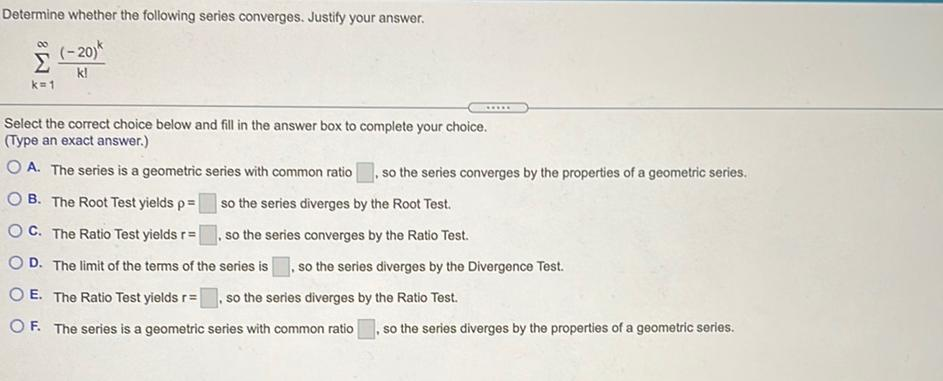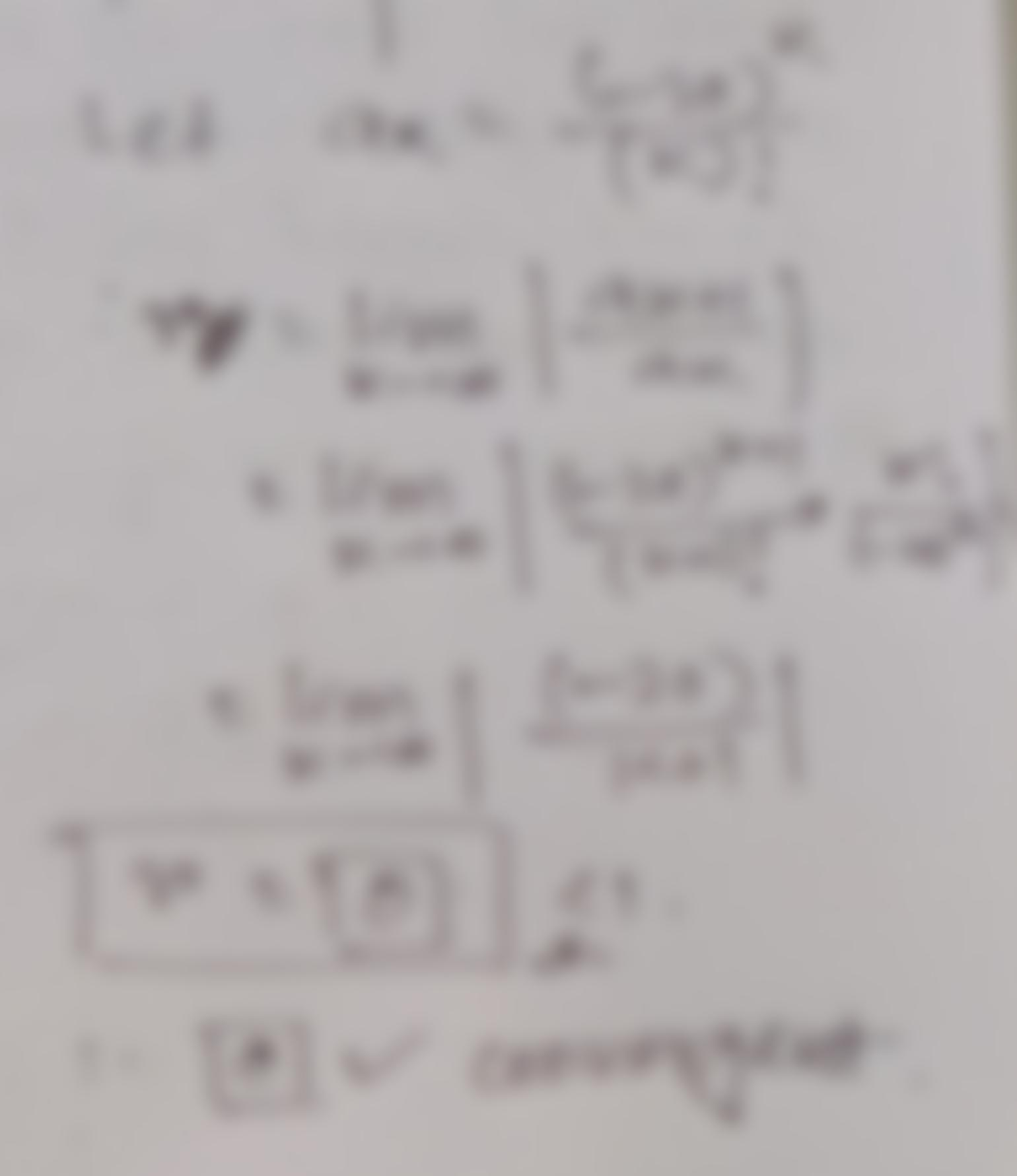Question:

Determine whether the following series converges. Justify your answer. (-20) k! k=1 È Select the correct choice below and fill iDetermine whether the following series converges. Justify your answer. (-20) k! k=1 È Select the correct choice below and fill in the answer box to complete your choice. (Type an exact answer.) O A. The series is a geometric series with common ratio , so the series converges by the properties of a geometric series. OB. The Root Test yields p= so the series diverges by the Root Test. OC. The Ratio Test yields r= so the series converges by the Ratio Test. OD. The limit of the terms of the series is so the series diverges by the Divergence Test. O E. The Ratio Test yields r= so the series diverges by the Ratio Test. OF. The series is a geometric series with common ratio , so the series diverges by the properties of a geometric series.